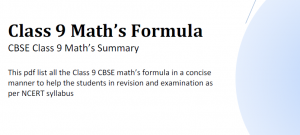## Download Maths Formulas pdf Class 9 CBSEThis article is about CBSE Maths Formulas pdf Class 9. Having all the formula in one place is always helpful for the students, Keeping that in mind,We have prepared a Maths formula pdf for CBSE Class 9 students.  This math formula pdf contains the maths formulas that are aimed for class 9 students as per the NCERT math book. Chapters covered in this file are

1. Polynomial
2. Coordinate Geometry
3. Euclid Geometry
4. Line and Angles
5. Triangles
7. Area of Parallelogram
8. Circles
9. Linear equation in two variable
10. Statistics
11. Probability
12. Heron Formula
13. Surface Area and Volume

Other important class 9 pages where you can find science and maths study material are
[standout-css3-button href=”https://physicscatalyst.com/class9.php”]Class 9 Science[/standout-css3-button][standout-css3-button href=”https://physicscatalyst.com/class9_maths.php”]Class 9 maths[/standout-css3-button]
We really hope that this CBSE Maths Formula pdf will help students in the revision for their examination. We have prepared this file keeping CBSE syllabus in mind.

We would like to hear from you and get your suggestions about what you like and what else you want to have.

### Search Our Website

Subscribe
Notify of

This site uses Akismet to reduce spam. Learn how your comment data is processed.